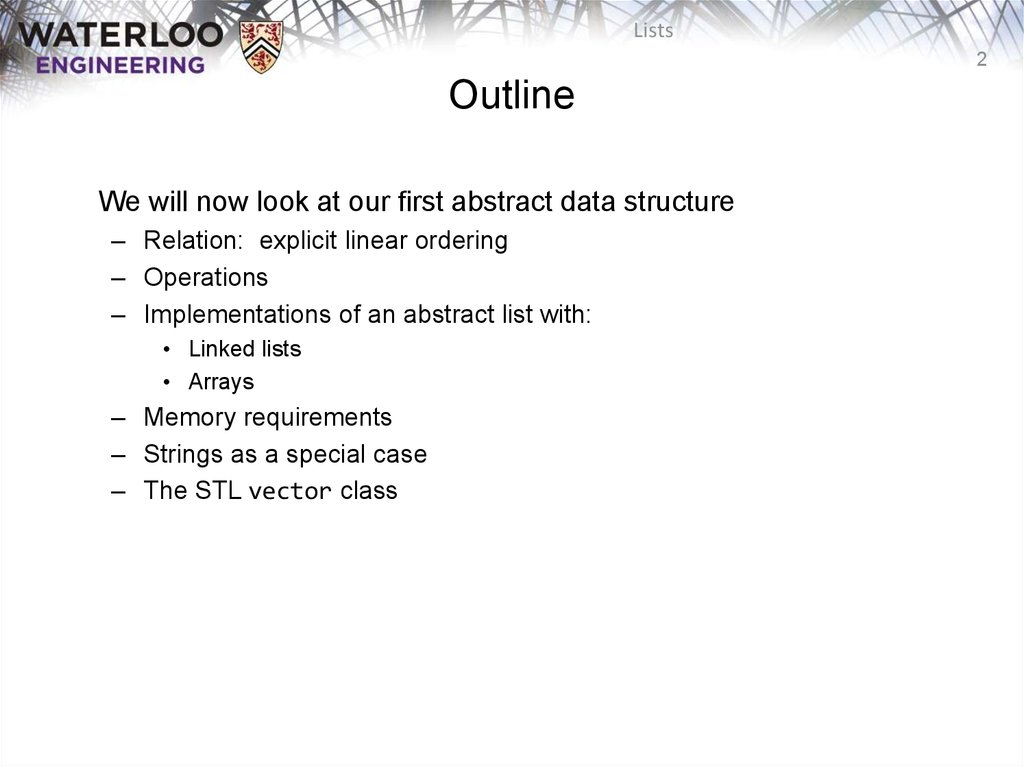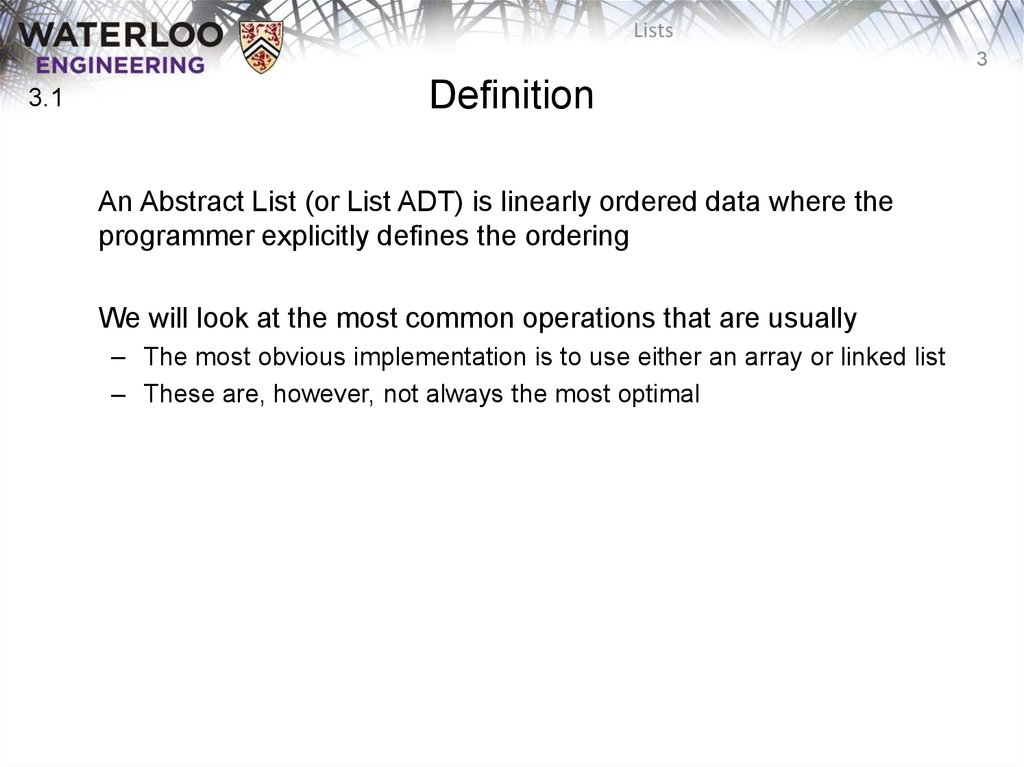# ECE 250. Algorithms and Data Structures. Lists

## 1.

ECE 250 Algorithms and Data Structures
Lists
Douglas Wilhelm Harder, M.Math. LEL
Department of Electrical and Computer Engineering
University of Waterloo
ece.uwaterloo.ca
[email protected]
© 2006-2013 by Douglas Wilhelm Harder. Some rights reserved.

## 2. Outline

Lists
2
Outline
We will now look at our first abstract data structure
– Relation: explicit linear ordering
– Operations
– Implementations of an abstract list with:
• Arrays
– Memory requirements
– Strings as a special case
– The STL vector class

## 3. Definition

Lists
3
3.1
Definition
An Abstract List (or List ADT) is linearly ordered data where the
programmer explicitly defines the ordering
We will look at the most common operations that are usually
– The most obvious implementation is to use either an array or linked list
– These are, however, not always the most optimal

## 4. Operations

Lists
4
Operations
3.1.1
Operations at the kth entry of the list include:
Insertion of a new object
Erasing an object
Replacement of the object

## 5. Operations

Lists
5
3.1.1
Operations
next object
Given two abstract lists, we may want to
– Concatenate the two lists
– Determine if one is a sub-list of the other

## 6. Locations and run times

Lists
6
3.1.2
Locations and run times
The most obvious data structures for implementing an abstract list
– We will review the run time operations on these structures
We will consider the amount of time required to perform actions
such as finding, inserting new entries before or after, or erasing
entries at
– the first location (the front)
– an arbitrary (kth) location
– the last location (the back or nth)
The run times will be Q(1), O(n) or Q(n)

Lists
7
3.1.3
We will consider these for

Lists
8
3.1.3.1
Find
Insert Before
Insert After
Replace
Erase
Next
Previous
* These
Front/1st node
Q(1)
Q(1)
Q(1)
Q(1)
Q(1)
Q(1)
n/a
kth node
O(n)*
O(n)*
Q(1)*
Q(1)*
O(n)*
Q(1)*
O(n)*
Back/nth node
Q(1)
Q(n)
Q(1)
Q(1)
Q(n)
n/a
Q(n)
assume we have already accessed the kth entry—an O(n) operation

Lists
9
3.1.3.1
Find
Insert Before
Insert After
Replace
Erase
Next
Previous
Front/1st node
Q(1)
Q(1)
Q(1)
Q(1)
Q(1)
Q(1)
n/a
kth node
O(n)*
Q(1)*
Q(1)*
Q(1)*
Q(1)*
Q(1)*
O(n)*
Back/nth node
Q(1)
Q(1)
Q(1)
Q(1)
Q(n)
n/a
Q(n)
By replacing the value in the node in question, we can speed things up
– useful for interviews

Lists
10
3.1.3.2
Find
Insert Before
Insert After
Replace
Erase
Next
Previous
* These
Front/1st node
Q(1)
Q(1)
Q(1)
Q(1)
Q(1)
Q(1)
n/a
kth node
O(n)*
Q(1)*
Q(1)*
Q(1)*
Q(1)*
Q(1)*
Q(1)*
Back/nth node
Q(1)
Q(1)
Q(1)
Q(1)
Q(1)
n/a
Q(1)
assume we have already accessed the kth entry—an O(n) operation

Lists
11
3.1.3.2
Accessing the kth entry is O(n)
Insert Before
Insert After
Replace
Erase
Next
Previous
kth node
Q(1)
Q(1)
Q(1)
Q(1)
Q(1)
Q(1)

## 12. Other operations on linked lists

Lists
12
3.1.3.3
Other operations on linked lists include:
– Allocation and deallocating the memory requires Q(n) time
– Concatenating two linked lists can be done in Q(1)
• This requires a tail pointer

## 13. Arrays

Lists
13
3.1.4
Arrays
We will consider these operations for arrays, including:
– Standard or one-ended arrays
– Two-ended arrays

## 14. Standard arrays

Lists
14
3.1.4
Standard arrays
We will consider these operations for arrays, including:
– Standard or one-ended arrays
– Two-ended arrays

## 15. Run times

Lists
15
Run times
Accessing
the kth entry
Arrays
Two-ended arrays
O(n)
Q(1)
Insert or erase at the
Front
kth entry
Q(1)
Q(1)*
Q(n)
Q(1)
O(n)
Back
Q(1) or Q(n)
Q(1)
Q(1)
* Assume we have a pointer to this node

## 16. Data Structures

Lists
16
Data Structures
In general, we will only use these basic data structures if we can
restrict ourselves to operations that execute in Q(1) time, as the only
alternative is O(n) or Q(n)
Interview question: in a singly linked list, can you speed up the two
O(n) operations of
– Inserting before an arbitrary node?
– Erasing any node that is not the last node?
If you can replace the contents of a node, the answer is “yes”
– Replace the contents of the current node with the new entry and insert
after the current node
– Copy the contents of the next node into the current node and erase the
next node

## 17. Memory usage versus run times

Lists
17
Memory usage versus run times
All of these data structures require Q(n) memory
– Using a two-ended array requires one more member variable, Q(1), in
order to significantly speed up certain operations
– Using a doubly linked list, however, required Q(n) additional memory to
speed up other operations

## 18. Memory usage versus run times

Lists
18
Memory usage versus run times
As well as determining run times, we are also interested in memory
usage
In general, there is an interesting relationship between memory and
time efficiency
For a data structure/algorithm:
– Improving the run time usually
requires more memory
– Reducing the required memory
usually requires more run time

## 19. Memory usage versus run times

Lists
19
Memory usage versus run times
Warning: programmers often mistake this to suggest that given any
solution to a problem, any solution which may be faster must require
more memory
This guideline not true in general: there may be different data
structures and/or algorithms which are both faster and require less
memory
– This requires thought and research

## 20. The sizeof Operator

Lists
20
The sizeof Operator
In order to determine memory usage, we must know the memory
usage of the various built-in data types and classes
– The sizeof operator in C++ returns the number of bytes occupied by a
data type
– This value is determined at compile time
• It is not a function

## 21. The sizeof Operator

Lists
21
The sizeof Operator
#include <iostream>
using namespace std;
int main() {
cout <<
cout <<
cout <<
cout <<
cout <<
cout <<
cout <<
cout <<
"bool
"char
"short
"int
"char *
"int *
"double
"int
return 0;
}
"
"
"
"
"
"
"
"
<<
<<
<<
<<
<<
<<
<<
<<
sizeof(
sizeof(
sizeof(
sizeof(
sizeof(
sizeof(
sizeof(
sizeof(
bool )
char )
short )
int )
char * )
int * )
double )
int )
<<
<<
<<
<<
<<
<<
<<
<<
endl;
endl;
endl;
endl;
endl;
endl;
endl;
endl;
{eceunix:1} ./a.out # output
bool
1
char
1
short
2
int
4
char *
4
int *
4
double
8
int
40
{eceunix:2}

## 22. Abstract Strings

Lists
22
Abstract Strings
A specialization of an Abstract List is an Abstract String:
– The entries are restricted to characters from a finite alphabet
– This includes regular strings “Hello world!”
The restriction using an alphabet emphasizes specific operations
that would seldom be used otherwise
– Substrings, matching substrings, string concatenations
It also allows more efficient implementations
– String searching/matching algorithms
– Regular expressions

## 23. Abstract Strings

Lists
23
Abstract Strings
Strings also include DNA
– The alphabet has 4 characters: A, C, G, and T
– These are the nucleobases:
Bioinformatics today uses many of the
computer science:
– Dan Gusfield, Algorithms on Strings, Trees and Sequences: Computer
Science and Computational Biology, Cambridge, 1997
– References:
http://en.wikipedia.org/wiki/DNA
http://en.wikipedia.org/wiki/Bioinformatics

## 24. Standard Template Library

Lists
24
Standard Template Library
In this course, you must understand each data structure and their
associated algorithms
– In industry, you will use other implementations of these structures
The C++ Standard Template Library (STL) has an implementation of
the vector data structure
– Excellent reference:
http://www.cplusplus.com/reference/stl/vector/

## 25. Standard Template Library

Lists
25
Standard Template Library
#include <iostream>
#include <vector>
using namespace std;
int main() {
vector<int> v( 10, 0 );
cout << "Is the vector empty?
cout << "Size of vector: "
" << v.empty() << endl;
<< v.size() << endl;
v = 42;
v = 91;
for ( int k = 0; k < 10; ++k ) {
cout << "v[" << k << "] = " << v[k] << endl;
}
return 0;
}
\$ g++ vec.cpp
\$ ./a.out
Is the vector empty?
Size of vector: 10
v = 42
v = 0
v = 0
v = 0
v = 0
v = 0
v = 0
v = 0
v = 0
v = 91
\$
0

## 26. Summary

Lists
26
Summary
In this topic, we have introduced Abstract Lists
– Explicit linear orderings
– Implementable with arrays or linked lists
• Each has their limitations
• Introduced modifications to reduce run times down to Q(1)
– Discussed memory usage and the sizeof operator
– Looked at the String ADT
– Looked at the vector class in the STL

## 27. References

Lists
27
References


Donald E. Knuth, The Art of Computer Programming, Volume 1:
Fundamental Algorithms, 3rd Ed., Addison Wesley, 1997, §2.2.1, p.238.
Weiss, Data Structures and Algorithm Analysis in C++, 3rd Ed., Addison
Wesley, §3.3.1, p.75.

## 28. Usage Notes

Lists
28
Usage Notes
• These slides are made publicly available on the web for anyone to
use
• If you choose to use them, or a part thereof, for a course at another
institution, I ask only three things:
– that you inform me that you are using the slides,
– that you acknowledge my work, and
– that you alert me of any mistakes which I made or changes which you
make, and allow me the option of incorporating such changes (with an
acknowledgment) in my set of slides
Sincerely,
Douglas Wilhelm Harder, MMath
[email protected]